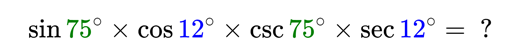Geometry

# Trigonometric Functions Problem Solving

$\theta$ is an acute angle such that $\tan (\theta) = \frac{1}{3}$. What is the value of $10 \sqrt{10}\cdot\left(\sin \theta + \cos \theta \right)$?Let $m$ and $M$ be the minimum and maximum values of the domain of $f(x) = \sin^{-1}(x^2 - 360)$, respectively. What is the value of $M - m$?

Details and assumptions

$\sin^{-1} x$ denotes the functional inverse of $\sin x$ not the reciprocal $\frac{1}{\sin x}.$

Let $O$ be the origin and $P$ be a point in the fourth quadrant on the x-y plane. Let $270 ^\circ < \theta < 360^\circ$ be an angle formed by $OP$ with the positive x-axis. Similarly $Q$ is a point in the fourth quadrant on the x-y plane where $|OQ| = |OP|$ and the angle formed by $OQ$ and the x-axis is $7\theta$. For what value of $\theta$ does the segments $OP$ and $OQ$ coincide?

Let $\theta$ be the angle between the x-axis and the line connecting the origin $O (0,0)$ and the point $P (-8,-15)$, where $180^\circ < \theta < 270^\circ$. Given that $\sin \theta + \cos \theta + \tan \theta = \frac{a}{b}$, where $a$ and $b$ are coprime positive integers. What is the value of $a+b$?

×